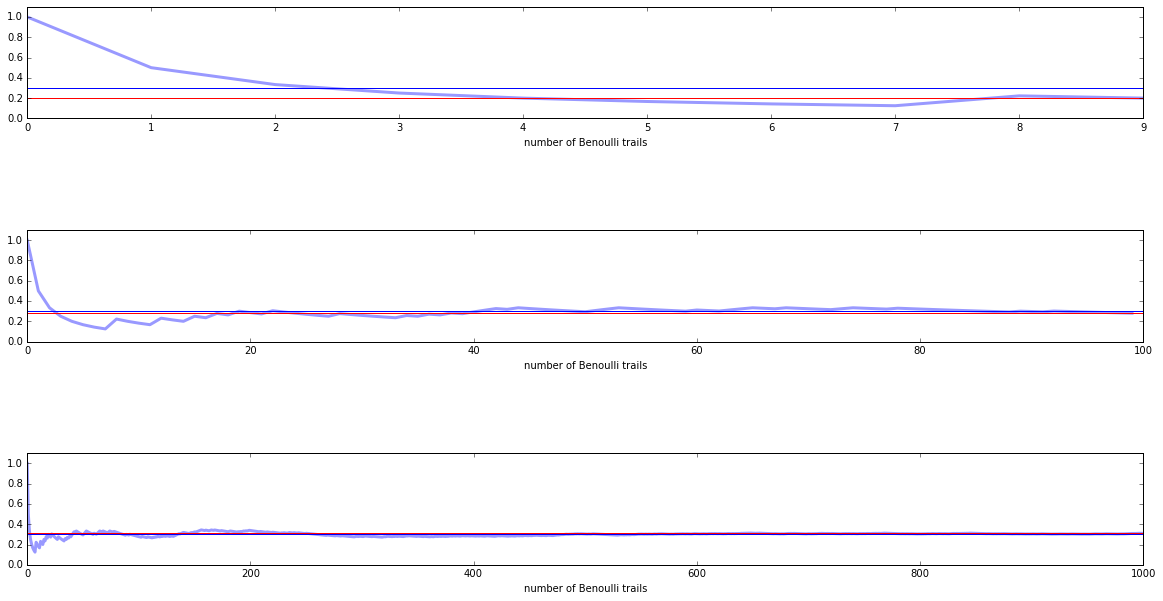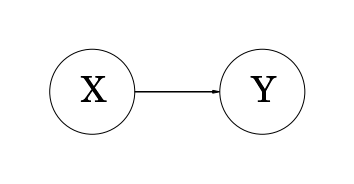maximum-likelihood-learning slides

## Parameter Learning by Maximum Likelihood¶

Examples:

• $n$-iid Bernoulli trails - Binomial distribution
• Simple Bayesian Network with two binary random variables

#### Bayes Rule for Data and Model Parameters¶

• $\mathcal D$: Data
• $\mathcal \theta$: Parameter of the model
$$p(\theta | \mathcal D) = \frac{p(\mathcal D | \theta) p(\theta)}{ p(\mathcal D)}$$
• numerator:
• first term: "likelihood"
• second term: "prior"
• denominator: "marginal likelihood" (or "evidence") $$posterior = \frac{likelihood \cdot prior}{evidence}$$

#### Likelihood¶

Likelihood function is considered as a function of $\theta$:

$$\mathcal L (\theta) = p(\mathcal D | \theta)$$

The likelihood function is not a probability function!

"Never say 'the likelihood of the data'. Alway say 'the likelihood of the parameters'. The likelihood function is not a probability distribution." (from D. MacKay: Information Theory, Inference and Learning Algorithms, Page 29, Cambride 2003, http://www.inference.phy.cam.ac.uk/itila/book.html)

## Maximum Likelihood¶

$$\arg\max_\theta \mathcal L(\theta)$$

often the negativ log-likelihood is minimized:

$$\arg\max_\theta \mathcal L(\theta) = \arg\min_\theta \left(- \mathcal \log \left( \mathcal L(\theta) \right) \right)$$

Example: Binomial Distribution (e.g. tossing a thumtack)

$$\arg\min_\theta \left( - \mathcal \log L(\theta) \right)= \arg\min_\theta - \log \left( {n \choose k} \theta^k (1-\theta)^{n-k}\right)$$

necessary condition for a minimum:

$$0 = \frac{d}{d\theta} \left( \theta^k (1-\theta)^{n-k}\right) = k \theta^{k-1} (1-\theta)^{n-k} - (n-k) \theta^{k} (1-\theta)^{n-k-1}$$$$k(1-\theta) = (n-k) \theta$$$$\theta_{ML} = \frac{k}{n}$$

Example: Thumbtack using maximum likelihood estimation.

In :
from IPython.display import Image
Image(filename='./thumbtack.jpg', width=200, height=200)

Out:In :
# number of iid flips
n = 14
from scipy.stats import bernoulli
bernoulli.rvs(0.3,size=n)

Out:
array([0, 0, 0, 0, 0, 0, 0, 0, 0, 1, 1, 0, 0, 0])

Sufficient statistics for $\mathcal D$ is $k$ (number of positive outcomes) and $n$ (number of total outcomes).

In :
def maximum_likelihood_estimate(theta, n_total):
r = bernoulli.rvs(theta, size=n_total)
mle=[np.sum(r[:i])/float(i) for i in range(1, len(r)+1)]
return r, mle

theta = 0.3

r, mle = maximum_likelihood_estimate(theta, n_total=10)
print "\nMaximum likelihood estimate for the parameter theta up to the k-th trail:"
mle

Maximum likelihood estimate for the parameter theta up to the k-th trail:

Out:
[0.0,
0.0,
0.0,
0.25,
0.40000000000000002,
0.33333333333333331,
0.42857142857142855,
0.375,
0.33333333333333331,
0.29999999999999999]

#### Law of large numbers¶

In :
plot_estimates(theta)### MLE for Bayesian Networks¶

Example Graph of two binary random variables $X$ and $Y$:

In :
draw_XY(3.)$\mathcal D$ consists of $n$ different observations:

• freq(X=0, Y=0) = $f$
• freq(X=0, Y=1) = $g$
• freq(X=1, Y=0) = $h$
• freq(X=1, Y=1) = $i$

with

• total number of observations: $n = f + g + h + i$
• number of $(X=1)$-observations: $k = h + i$
• number of $(X=0)$-observations: $l = f+g = n-k$
$$P(X, Y) = P(X) P(Y | X)$$

We have three free parameters for three Binomial distributions:

• $\theta_X$ : Probability of $X=1$
• $\theta_{0Y}$: Probability of $Y=1$ under the constraint $X=0$
• $\theta_{1Y}$: Probability of $Y=1$ under the constraint $X=1$

Joinly written as parameter vector $\vec \theta = (\theta_X, \theta_{0Y},\theta_{1Y})$ or as a set $\theta = \{\theta_X, \theta_{0Y},\theta_{1Y}\}$

The likelihood of parameter vector $\vec \theta = (\theta_X, \theta_{0Y},\theta_{1Y})$ given $\mathcal D$ is:

$$\mathcal L(\vec \theta) = p( \mathcal D| \vec \theta) = P(\mathcal D_X | \theta_X) P(\mathcal D_{Y|X} | \theta_{0Y}, \theta_{1Y})$$

Under omitting the constant (Binomial coeffients - given data):

$$\mathcal L(\vec \theta) \propto \left( \theta_X^{k} (1-\theta_X)^{n-k} \right) \left( \theta_{1Y}^{i} (1-\theta_{1Y})^{k-i} \right)^k \left( \theta_{0Y}^{g} (1-\theta_{0Y})^{l-g} \right)^{l}$$

The likelihood decomposes into a product of terms; that's is called Decomposability.

respectivly for the log-likelihood:

\begin{align} \log \mathcal L(\vec \theta) \propto & { } \left( k \log \theta_X + (n-k) \log (1-\theta_X) \right) + \\ & { } k \left( i \log \theta_{1Y} + (k-i) \log (1-\theta_{1Y}) \right) + \\ & { } l \left( g \log \theta_{0Y} + (l-g) \log (1-\theta_{0Y}) \right) \end{align}

So we have three independent terms \begin{align} \log \mathcal L(\vec \theta) \propto & { }\log \mathcal L(\theta_X) + \\ & { } \log \mathcal L(\theta_{1Y}) + \\ & { } \log \mathcal L(\theta_{0Y}) \end{align}

So searching for the argmax of $\log \mathcal L(\vec \theta)$ can be done by finding the argmax of each term independently. Also note that the leading $k$ and $l$ are just constants in the second resp. third term which can be neglected in the optimization.

Log-Likelihood of the first term:

$$\log \mathcal L (\theta_{X}) \propto k \log \theta_{X} + (n-k) \log (1-\theta_{X})$$

We already know how to compute (see above) the MLE (maximum likelihood estimator) of $\theta_{X}$:

$$\theta_{X}^{ML} = \frac{k}{n}$$

(Local) Log-Likelihood (second term):

$$\log \mathcal L(\theta_{1Y})\propto i \log \theta_{1Y} + (k-i) \log (1-\theta_{1Y})$$



MLE (maximum likelihood estimator):

$$\theta_{1Y}^{ML} = \frac{i}{k}$$

(Local) Log-Likelihood (third term):

$$\log \mathcal L(\theta_{0Y}) \propto g \log \theta_{0Y} + (l-g) \log (1-\theta_{0Y})$$



MLE (maximum likelihood estimator):

$$\theta_{0Y}^{ML} = \frac{g}{l}$$

So for (local) Binomial (and analog for Multinomials) the MLEs can be obtained just by ratios of frequencies.

#### Global decomposition:¶

• likelihood function decomposes as a product of independent terms for each CPD